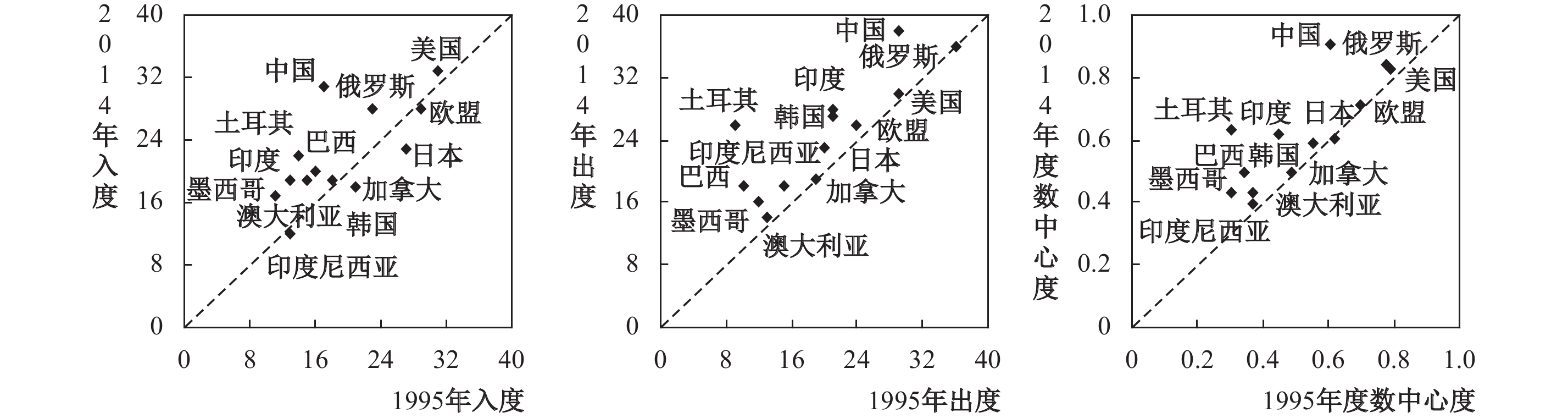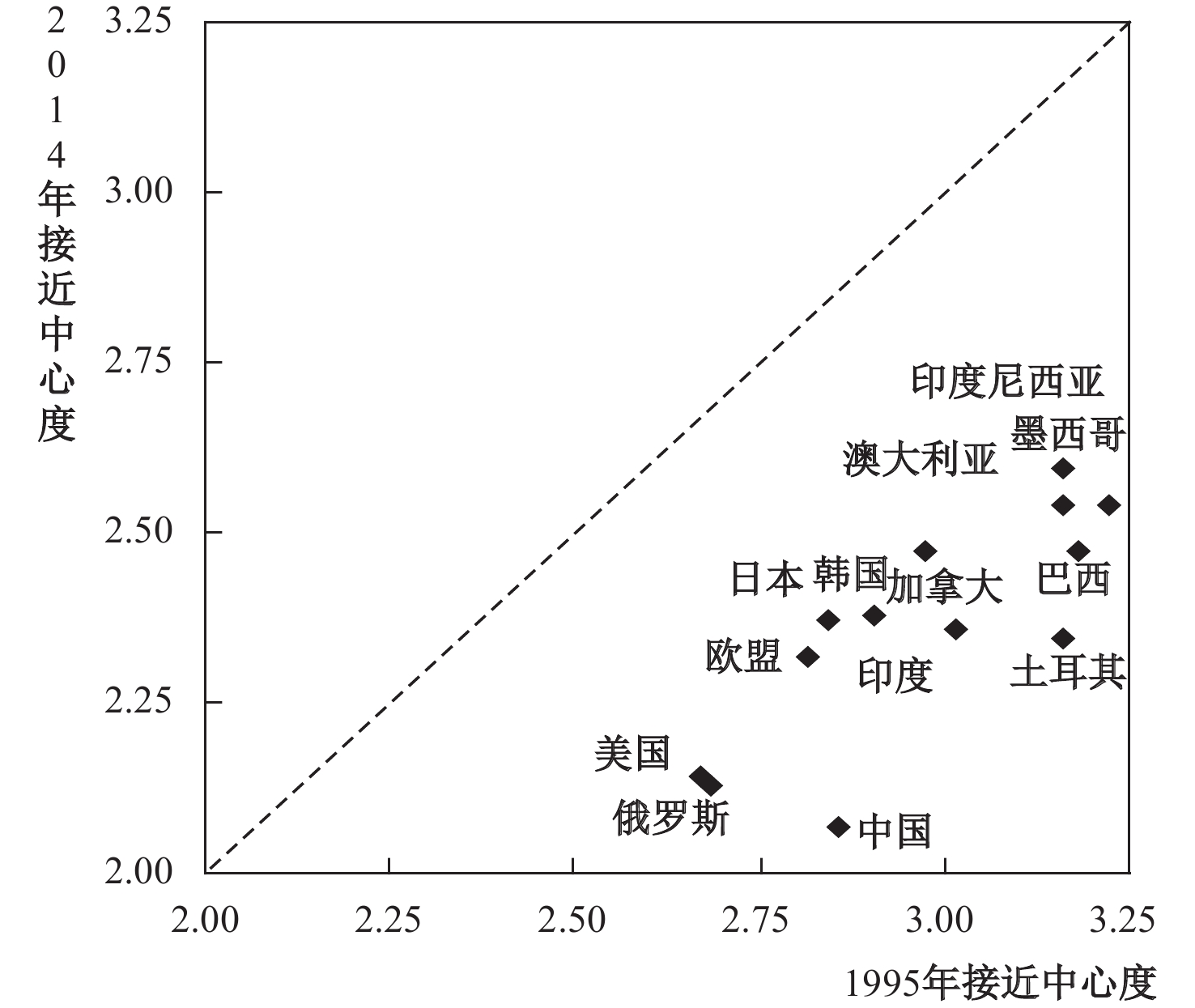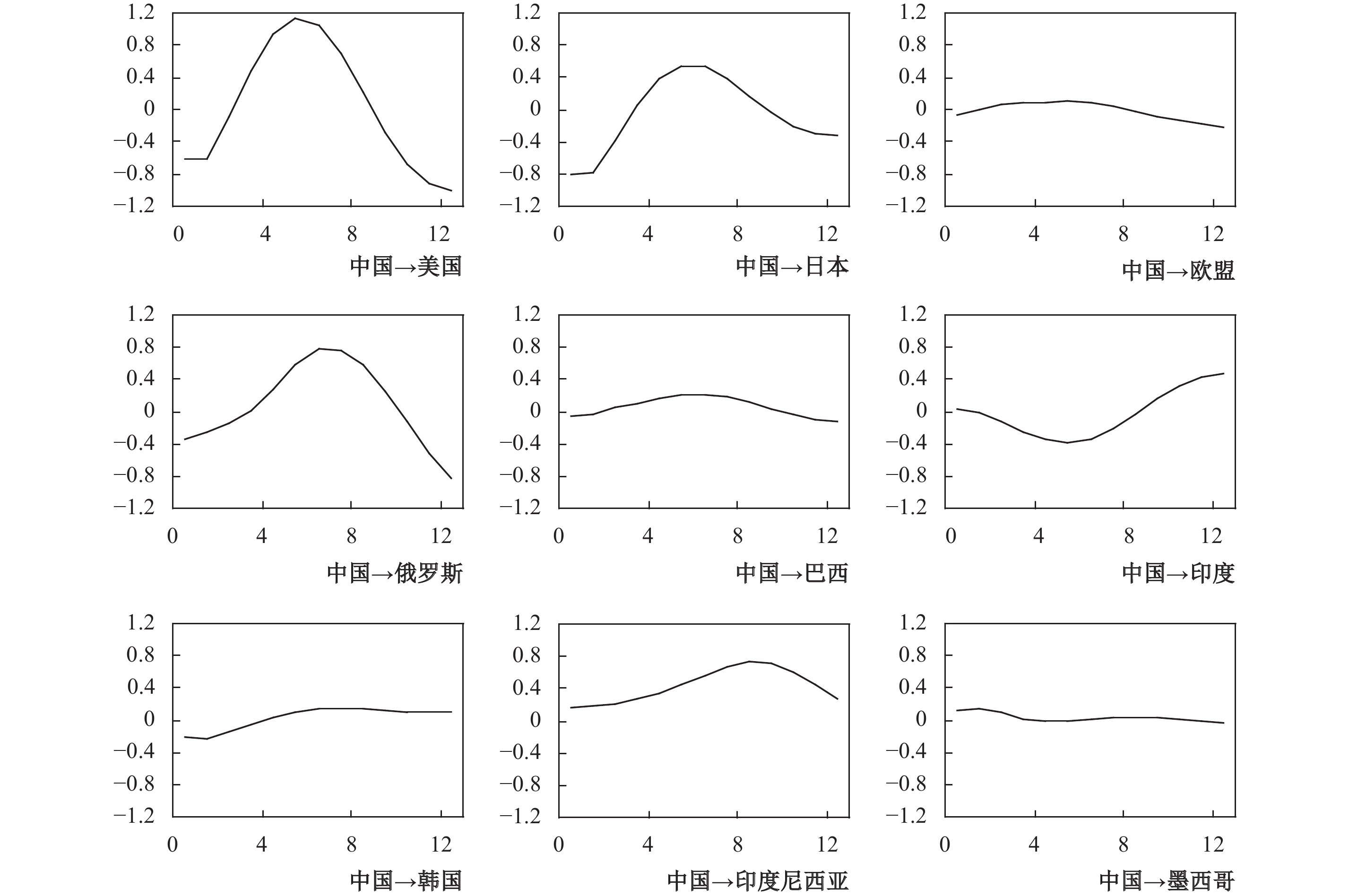﻿ “国际贸易—碳排放”网络的结构特征与传导路径研究《财经研究》
2019第45卷第3期
“国际贸易—碳排放”网络的结构特征与传导路径研究

A Research on the Structural Characteristics and Transmission Path of the “International Trade-Carbon Emission” Network
Zhang Tongbin, Sun Jing
School of Economics，Dongbei University of Finance and Economics，Dalian 116025，China
Summary: In this paper, we use the social network method to analyze the structure of the international carbon emission network, which provides new perspectives and research methods for the study of carbon emission relations among countries. Based on the association matrix of carbon emissions among countries, we specify weight matrices and embed them into the global vector auto-regression (GVAR) model, which makes a more accurate measurement of the relationship between economic growth and carbon emissions among countries, and deeply investigate the internal relationship between international economic growth and carbon emissions.　　Firstly, the structural characteristics of individuals in the carbon emission network are analyzed. The results show that in the international carbon emission network, developed economies have achieved carbon transfer through importing a large number of intermediate products, and their in-degree in the international carbon emission network is large. Comparatively, the BRIC countries and the emerging economies have received carbon imports from developed countries while exporting products, resulting in their high out-degree. Developed countries have achieved " products import and carbon export”, while developing countries have shown the characteristics of " products export and carbon import”. China has established a large number of production links with other countries through processing trade and other ways, and then the carbon emission links have been established and become increasingly close. In general, the centrality of developed economies is relatively stable in the international carbon emission network, while the degree of centrality in developing countries or emerging economies has increased significantly.　　Based on the network structure characteristic analysis of the international carbon emission matrix, we further explore the impacts of one country’s economic growth on other countries’ carbon emissions through the carbon emission network transmission from the perspective of network association. The results show that under the shock of China’s economic growth, the changes of carbon emissions of representative economies, except India, show inverted " U” shape characteristics. The economic growth of developed countries except Japan and BRIC countries has positive effects of inverted " U” type on China’s carbon emissions while the economic outputs of emerging economies exert " U” type negative impacts on the carbon emissions of China. The status of international industrial specialization and the structure of commodity exports can largely explain the shock differences of economic growth and carbon emissions between representative economies and China.
Key words: international carbon emissions    carbon transfer    network structure    transmission path    global vector auto-regression model

（一）国际碳排放矩阵的构建。为表述世界各国之间经济增长与碳排放的紧密关联，参照Chang和Shih（2005）、Montresor和Marzetti（2009）的研究，本文基于国际多区域投入产出（International Multi-Region Input-OutputIMRIO）表和各国碳排放量数据构建国家间“国际贸易—碳排放”矩阵，简称国际碳排放矩阵。国际多区域投入产出表如式（1）所示。

 $\left( {\begin{array}{*{20}{c}} {{x_1}} \\ \vdots \\ {{x_n}} \end{array}} \right){\rm{ = }}\left( {\begin{array}{*{20}{c}} {{a_{11}}}& \cdots &{{a_{1n}}} \\ \vdots & \ddots & \vdots \\ {{a_{n1}}}& \cdots &{{a_{nn}}} \end{array}} \right)\left( {\begin{array}{*{20}{c}} {{x_1}} \\ \vdots \\ {{x_n}} \end{array}} \right) + \left( {\begin{array}{*{20}{c}} {\sum\nolimits_{j = 1}^n {{y_{1j}}} } \\ \vdots \\ {\sum\nolimits_{j = 1}^n {{y_{nj}}} } \end{array}} \right)$ (1)

 $P = \left( {\hat c} \right){\left( {\hat x} \right)^{ - 1}}{\left( {I - A} \right)^{ - 1}}Y$ (2)

 ${d_{ijt}} = \left\{ {\begin{array}{*{20}{c}} {1,\;\;\;{P_{ijt}} \geqslant m}\\ {0,\;\;\;{P_{ijt}} < m} \end{array}} \right.$ (3)

（二）网络结构分析方法。本文主要从刻画个体位置特征的中心性方面对国际碳排放网络的结构特征进行分析。根据中心国家对其他国家碳排放影响渠道的不同，中心度又可以划分为度数中心度、中间中心度及接近中心度三类。

1. 度数中心度：在国际碳排放网络中，一国的度数中心度指的是与该国家存在直接碳排放联系的国家数量，度数中心度越高，则该国与其他国家之间的联系越多，越处于网络的中心位置。每个国家的度数中心度可基于点入度和点出度计算得到，其中点入度指的是一国通过进口将本应属于该国的碳排放转移到其他国家的关系数量，点出度则是一国出口而为其他国家承担的碳排放，是其他国家转移到本国的关系数量。在二值化的国际碳排放矩阵中，第t年第i国家的点入度（IIndit）和点出度（IOutdit）的计算公式分别为式（4）和式（5）。

 $IIn{d_{it}} = \sum\nolimits_{j\left( {i \ne j} \right)} {{d_{jit}}} ,\;\;0 \leqslant IIn{d_{it}} \leqslant n - 1$ (4)
 $IOut{d_{it}} = \sum\nolimits_{j\left( {i \ne j} \right)} {{d_{ijt}}} ,\;\;0 \leqslant IOut{d_{it}}\leqslant n - 1$ (5)

 $I{D_{eit}} = {{\left( {IIn{d_{it}} + IOut{d_{it}}} \right)}/{\left( {2n - 2} \right)}}$ (6)

2. 中间中心度：中间中心度是反映一国在国际碳排放网络中“中介”或“桥梁”作用的指标，第t年国家i对于国家j和国家k而言的中间中心度为：

 ${b_{jkt}}\left( i \right) = {{{g_{jkt}}\left( i \right)}/{{g_{jkt}}}}$ (7)

 $I{C_{ABit}} = \sum\nolimits_j {\sum\nolimits_k {{b_{jkt}}\left( i \right)} } ,\;\;i \ne k \ne j,j < k$ (8)

3. 接近中心度：接近中心度利用国家间捷径的“距离”来表示一国在国际碳排放网络中的中心性，如果国家在碳排放网络中与其他国家的捷径“距离”都很短，则该国在网络中处于中心行动者位置。一国的接近中心度是国家与网络中其他国家的捷径距离之和，第t年国家i的接近中心度（ICAPit）定义为：

 $I{C_{APit}} = {{\left( {\sum\nolimits_j {{f_{jit}}} + \sum\nolimits_j {{f_{ijt}}} } \right)}/{\left( {2n - 2} \right)}}$ (9)图 1 代表性经济体的出入度与度数中心度图 2 代表性经济体的中间中心度图 3 代表性经济体的接近中心度

（一）GVAR模型的构建。参照张延群（2012）对全球向量自回归（GVAR）模型理论及方法的描述，本文设定了国际碳排放问题的GVAR模型，首先设定各个国家的VARX *模型为：

 ${X_{it}} = {\alpha _{i0}} + {\alpha _{i1}}t + {\varPhi _i}{X_{it - 1}}{\rm{ + }}{\varLambda _{i\,0}}X_{it}^* + {\varLambda _{i1}}X_{it - 1}^* + {\varepsilon _{it}}$ (10)

 $Y_{it}^{\rm{*}} = \sum\nolimits_{j = 0}^N {w_{ij}^Y{Y_{jt}}}$ (11)

 ${A_i}{Z_{it}} = {\alpha _{i0}} + {\alpha _{i1}}t + {B_i}{Z_{it - 1}} + {\varepsilon _{it}}$ (12)

 ${Z_{it}} = {W_i}{X_t}$ (13)

 ${A_i}{W_i}{X_t} = {\alpha _{i0}} + {\alpha _{i1}}t + {B_i}{W_i}{X_{t - 1}} + {\varepsilon _{it}}$ (14)

 $G{X_t} = {\alpha _0} + {\alpha _1}t + H{X_{t - 1}} + {\varepsilon _t}$ (15)

 ${X_{it}} = {\alpha _{i0}} + {\alpha _{i1}}t + {\varPhi _i}{X_{it - 1}} + {\varLambda _{i0}}X_{it}^* + {\varLambda _{i1}}X_{it - 1}^* + {\psi _{i0}}{d_t} + {\psi _{i1}}{d_{t - 1}} + {\varepsilon _{it}}$ (16)

 $G{X_t} = {\alpha _0} + {\alpha _1}t + H{X_{t - 1}} + {\psi _0}{d_t} + {\psi _1}{d_{t - 1}} + {\varepsilon _t}$ (17)

（二）经济增长对碳排放的影响路径分析。广义脉冲响应函数（Generalized Impulse Response FunctionGIRF）是全球向量自回归模型中的一种重要方法，能够反映模型中经济产出变量与碳排放变量之间的动态冲击效应。本文对GVAR模型进行估计后，采用GIRF方法对中国与代表性经济体经济增长和碳排放的影响关系进行分析，一方面研究中国经济增长对其他经济体碳排放的冲击效应，另一方面分析其他国家和地区经济增长对中国碳排放的影响效应。为简化起见，本文仅选取了与中国经济联系最为紧密的9个代表性经济体进行分析，按照经济体类型可以划分为发达国家、金砖国家及部分新兴经济体国家三组，其中发达经济体包括美国、日本、欧盟；金砖国家包括俄罗斯、巴西、印度；部分新兴经济体国家包括韩国、印度尼西亚、墨西哥。

1. 中国经济增长对代表性经济体碳排放的冲击效应与传导路径。对中国实际GDP进行一个标准差的正向冲击，得到9个代表性经济体碳排放量的响应函数值，结果如图4所示。图 4 中国经济增长对代表性经济体碳排放的影响

2. 代表性经济体产出增长对中国碳排放的影响效应与传导路径。本文分别对9个代表性经济体的实际GDP进行一个标准差的正向冲击后，得到中国碳排放量的脉冲响应图形。图 5 代表性经济体产出增长对中国碳排放的影响

① 数据来源于《2014年中国对外贸易发展情况》，中华人民共和国商务部综合司，网址：http://zhs.mofcom.gov.cn/。